## Two identical loudspeakers 2.0 m apart are emitting sound waves into a room where the speed of sound is 340 m/sec. John is standing 5.0m in

Question

Two identical loudspeakers 2.0 m apart are emitting sound waves into a room where the speed of sound is 340 m/sec. John is standing 5.0m in front of one of the speakers, perpendicular to the line joining the speakers, and hears a maximum in the intensity of the sound. What is the lowest possible frequency of sound for which this is possible?

in progress 0
6 months 2021-07-18T01:02:07+00:00 1 Answers 25 views 0

1. Answer: The lowest possible frequency of sound for which this is possible is 212.5 Hz.

Explanation:

It is known that formula for path difference is as follows.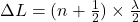… (1)

where, n = 0, 1, 2, and so on

As John is standing perpendicular to the line joining the speakers. So, the value of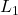is calculated as follows.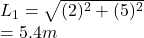Hence, path difference is as follows.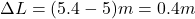For lowest frequency, the value of n = 0.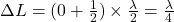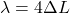where,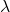= wavelength

The relation between wavelength, speed and frequency is as follows.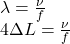where,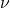= speed

f = frequency

Substitute the values into above formula as follows.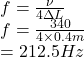Thus, we can conclude that the lowest possible frequency of sound for which this is possible is 212.5 Hz.